##### Geometry: 1,001 Practice Problems For Dummies (+ Free Online Practice)In geometry, the triangle inequality theorem states that when you add the lengths of any two sides of a triangle, their sum will be greater that the length of the third side.

By using the triangle inequality theorem and the exterior angle theorem, you should have no trouble completing the inequality proof in the following practice question.

## Practice questions

Complete the following proof by adding the missing statement or reason.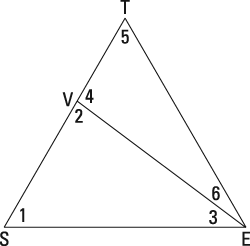Given: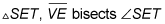Prove: ET > TV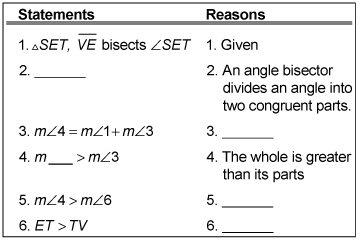1. What is the statement for Reason 2?

2. What is the reason for Statement 3?

3. What is the missing angle in Statement 4?

4. What is the reason for Statement 5?

5. What is the reason for Statement 6?

1.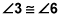A bisector divides an angle into two congruent angles.

2. The exterior angle of a triangle is equal to the sum of the two nonadjacent interior angles.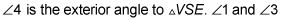are the two nonadjacent interior angles of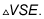The exterior angle of a triangle is equal to the sum of the two nonadjacent interior angles of the triangle; therefore,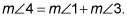3.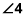You found thatThe whole is greater than its parts, which means that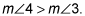4. Transitive property

Because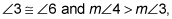the transitive property finds that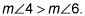5. In a triangle, the longest side is opposite the largest angle.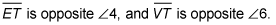You discovered thatIn a triangle, the longest side is opposite the largest angle, so ET > TV.